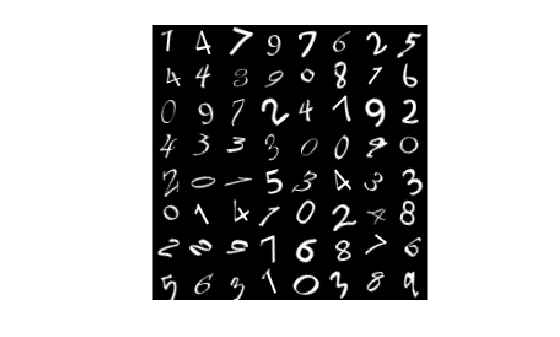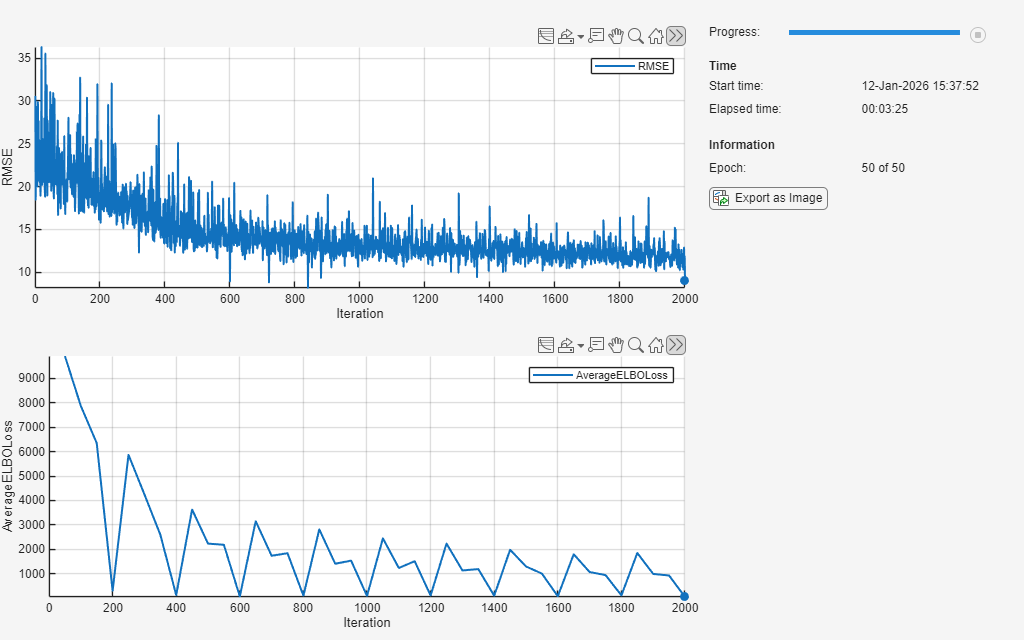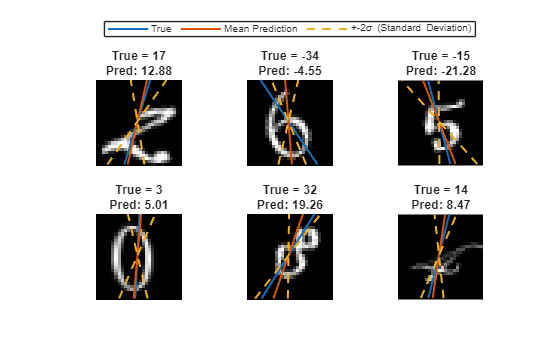# Train Bayesian Neural Network

This example shows how to train a Bayesian neural network (BNN) for image regression using Bayes by backpropagation . You can use a BNN to predict the rotation of handwritten digits and model the uncertainty of those predictions.

A Bayesian neural network (BNN) is a type of deep learning network that uses Bayesian methods to quantify the uncertainty in the predictions of a deep learning network. This example uses Bayes by backpropagation (also known as Bayes by backprop) to estimate the distribution of the weights of a neural network. By using a distribution of weights instead of a single set of weights, you can estimate the uncertainty of the network predictions.

This figure shows an example of the predicted angles of rotation and the uncertainty regions for an estimated distribution of weights.Load the digits data set. This data set contains synthetic images of handwritten digits together with the corresponding angles (in degrees) by which each image is rotated.

Load the training and test images as 4-D arrays using digitTrain4DArrayData and digitTest4DArrayData. The TTrain and TTest outputs are the rotation angles in degrees. The training and test data sets each contain 5000 images.

[XTrain,~,TTrain] = digitTrain4DArrayData; [XTest,~,TTest] = digitTest4DArrayData;

Create a single datastore that contains the training predictors and responses. To convert numeric arrays to datastores, use arrayDatastore. Then, use the combine function to combine these datastores into a single datastore.

dsXTrain = arrayDatastore(XTrain,IterationDimension=4); dsTTrain = arrayDatastore(TTrain); dsTrain = combine(dsXTrain,dsTTrain);

Extract the size of the responses and the number of observations.

numResponses = size(TTrain,2)
numResponses = 1 
numObservations = numel(TTrain)
numObservations = 5000 

Display 64 random training images.

idx = randperm(numObservations,64); I = imtile(XTrain(:,:,:,idx)); figure imshow(I)### Define Network Architecture

To model the weights and biases using a distribution rather than a single deterministic set, you must define a probability distribution for the weights. You can define the distribution using Bayes' theorem:

$\mathit{P}\left(\mathrm{parameters}\text{\hspace{0.17em}}|\mathrm{data}\right)=\frac{\mathit{P}\left(\mathrm{data}|\mathrm{parameters}\right)×\mathit{P}\left(\mathrm{parameters}\right)}{\mathit{P}\left(\mathrm{data}\right)}\text{\hspace{0.17em}}\propto \mathrm{likelihood}×\mathrm{prior}$

where $\mathit{P}\left(\mathrm{data}|\mathrm{parameters}\right)=L\left(\mathrm{parameters}|\mathrm{data}\right)$ is the likelihood and $P\left(\mathrm{parameters}\right)$ is the prior distribution. In this example, you set the weights and biases to follow a Gaussian distribution (corresponding to squared-loss). During training, the network learns the means and variances of the Gaussian distributions, which determine the distributions of the weights and biases.

Set the prior to a Gaussian mixture model  with two components, each with a mean of 0 and variances sigma1 and sigma2. You can fix the variances before training or learn them during training. Both components of the mixture model have a mixing proportion of 0.5.

Define a Bayesian neural network for image regression.

• For image input, specify an image input layer with an input size matching the training data.

• Do not normalize the image input. Set the Normalization option of the input layer to "none".

• Specify three Bayes fully connected layers with ReLU activation layers between them.

A Bayes fully connected layer is a type of fully connected layer that stores the average weights and biases of the expected distribution of the weights. When computing the activations of the layer, the software shifts the mean weights and biases by random Gaussian noise and uses the shifted weights and biases to compute the outputs of the layer.

To create a Bayes fully connected layer, use the bayesFullyConnectedLayer.m custom layer, attached to this example as a supporting file. The Bayes fully connected layer takes as input the output size and the parameters of the prior probabilities of the weight distribution, sigma1 and sigma2.

Define the network.

inputSize = [28 28 1]; outputSize = 784; sigma1 = 1; sigma2 = 0.5; layers = [ imageInputLayer(inputSize,Normalization="none") bayesFullyConnectedLayer(outputSize,Sigma1=sigma1,Sigma2=sigma2) reluLayer bayesFullyConnectedLayer(outputSize/2,Sigma1=sigma1,Sigma2=sigma2) reluLayer bayesFullyConnectedLayer(1,Sigma1=sigma1,Sigma2=sigma2)]; 

Create a dlnetwork object from the layer array.

net = dlnetwork(layers);

Visualize the network using analyzeNetwork. You can see that the learnables for the Bayes fully connected layers include the means and variances for the weights and biases.

analyzeNetwork(net)#### Define Learnable Parameters

The learnable parameters comprise network (layer) learnables and global learnables. During training, the algorithm updates these learnable parameters:

• The means and variances of the layer weights and biases (per layer)

• The prior probabilities for the weight distributions (per layer)

• The sampling noise (global)

#### Initialize Sampling Noise

Use sampling noise to represent the noise in the predictions of the neural network. Learn the sampling noise with the network weights and biases.

Initialize the sampling noise.

samplingNoise = dlarray(1);

#### Initialize Prior Probability

You can fix the prior variance parameters or learn them during training like the other learnable parameters. Learn the prior parameters during training using a small learn rate so that their values remain close to the initial values. Set the initial learn rate to 0.25.

doLearnPrior = true; priorLearnRate = 0.25; numLearnables = size(net.Learnables,1); for i=1:numLearnables layerName = net.Learnables.Layer(i); parameterName = net.Learnables.Parameter(i); if parameterName == "Sigma1" || parameterName == "Sigma2" if doLearnPrior net = setLearnRateFactor(net,layerName,parameterName,priorLearnRate); else net = setLearnRateFactor(net,layerName,parameterName,0); end end end

### Define Model Loss Functions

Define a function that returns the model loss and the gradients of the loss with respect to the learnable parameters. In this example, you minimize the evidence lower bound (ELBO) loss defined in the Evidence Lower Bound Loss section.

Create the function modelLoss, listed in the Model Loss Function section. The function takes as input a dlnetwork object and a mini-batch of input data with corresponding targets. The function returns these values:

• ELBO loss

• Root mean squared error (RMSE)

• Gradients of the loss with respect to the learnable parameters

• Gradients of the loss with respect to the sampling noise

• Network state

### Specify Training Options

Train for 50 epochs with a mini-batch size of 128.

numEpochs = 50; miniBatchSize = 128;

Track the ELBO loss during training. Plot the loss every 50 iterations and average the loss across five samples of the learnable parameters.

numSamplesForavgELBO = 5; averageLossComputationFrequency = 50;

### Train Model

Create a minibatchqueue object to process and manage the mini-batches of images. For each mini-batch:

• Use the custom mini-batch preprocessing function preprocessMiniBatch (defined at the end of this example) to one-hot encode the class labels.

• Format the image data with the dimension labels "SSCB" (spatial, spatial, channel, batch). By default, the minibatchqueue object converts the data to dlarray objects with underlying type single.

• Train on a GPU if one is available. By default, the minibatchqueue object converts each output to a gpuArray object if a GPU is available. Using a GPU requires a Parallel Computing Toolbox™ license and a supported GPU device. For information on supported devices, see GPU Computing Requirements (Parallel Computing Toolbox).

mbq = minibatchqueue(dsTrain, ... MiniBatchSize=miniBatchSize, ... MiniBatchFcn=@preprocessMiniBatch, ... MiniBatchFormat=["SSCB" "CB"]);

Initialize the parameters for Adam optimization.

trailingAvg = []; trailingAvgSq = []; trailingAvgNoise = []; trailingAvgNoiseSq = [];

Calculate the total number of iterations for the training progress monitor.

numIterationsPerEpoch = ceil(numObservations/miniBatchSize); numIterations = numEpochs*numIterationsPerEpoch;

Initialize the training progress monitor.

monitor = trainingProgressMonitor( ... Metrics=["RMSE","AverageELBOLoss"], ... Info="Epoch", ... XLabel="Iteration");

Train the model using a custom training loop. For each epoch, shuffle the data and loop over mini-batches of data. At the end of each iteration, display the training progress. For each mini-batch:

• Evaluate the model loss and gradients using dlfeval and the modelLoss function.

• Update the network parameters using the adamupdate function.

• Update the sampling noise parameters (global parameters) using the adamupdate function.

• Record the RMSE and the average ELBO loss.

iteration = 0; epoch = 0; % Loop over epochs. while epoch < numEpochs && ~monitor.Stop epoch = epoch + 1; miniBatchIdx = 0; % Shuffle data. shuffle(mbq); while hasdata(mbq) && ~monitor.Stop iteration = iteration + 1; miniBatchIdx = miniBatchIdx + 1; [X,T] = next(mbq); [elboLoss,rmsError,gradientsNet,gradientsNoise] = dlfeval(@modelLoss, ... net,X,T,samplingNoise,miniBatchIdx,numIterationsPerEpoch); % Update the network parameters using the Adam optimizer. [net,trailingAvg,trailingAvgSq] = adamupdate(net,gradientsNet, ... trailingAvg,trailingAvgSq,iteration); % Update the sampling noise. [samplingNoise,trailingAvgNoise,trailingAvgNoiseSq] = adamupdate(samplingNoise, ... gradientsNoise,trailingAvgNoise,trailingAvgNoiseSq,iteration); % Record the RMSE. recordMetrics(monitor,iteration,RMSE=double(rmsError)) % Record the average ELBO loss. if mod(iteration,averageLossComputationFrequency) == 0 avgELBOLoss = averageNegativeELBO(net,X,T,samplingNoise,miniBatchIdx, ... numIterationsPerEpoch,numSamplesForavgELBO); recordMetrics(monitor,iteration,AverageELBOLoss=double(avgELBOLoss)) end % Update the epoch and progress values in the monitor. updateInfo(monitor,Epoch=string(epoch) + " of " + string(numEpochs)) monitor.Progress = 100*(iteration/numIterations); end end### Test Network

BNNs learn the probability distribution of the weights rather than optimizing a single set of weights like convolutional neural networks. Therefore, you can view a BNN as an ensemble of networks where you sample each network from the learned probability distribution of the learnable parameters.

To test the accuracy of a BNN, generate $\mathit{N}$ samples for the weights and biases and compare the average prediction across the $\mathit{N}$ samples with the true value. The standard deviation across the $\mathit{N}$ predictions is the model uncertainty. Use the modelPosteriorSample function listed in the Model Prediction Function section to generate predictions for a set of inputs. The function samples $\mathit{N}$ times from the posterior distribution of the weights and biases. For each of the $\mathit{N}$ samples, the function generates predictions for the input images. The predictions from a BNN use a sample of the weights and biases; therefore, the predictions include some variational noise.

Convert the test data to a dlarray object.

XTest = dlarray(XTest,"SSCB"); if canUseGPU XTest = gpuArray(XTest); end

#### Test Single Image

Generate 10 samples for the first test image using modelPosteriorSample. The function returns 10 predictions for the angle of rotation. The final model prediction is the average value across the 10 predictions.

idx = 1; numSamples = 10; img = XTest(:,:,:,idx); predictions = modelPosteriorSample(net,img,samplingNoise,numSamples); YTestImg = mean(predictions,1);

Plot the true angle, the predicted angles, and the mean of the predictions.

figure lineWidth = 1.5; uncertaintyColor = "#EDB120"; I = extractdata(img); imshow(I,InitialMagnification=800) hold on inputSize = size(img,1); offset = inputSize/2; thetaActual = TTest(idx); plot(offset*[1 - tand(thetaActual),1 + tand(thetaActual)],[inputSize 0], ... LineWidth=lineWidth) thetaPredAvg = YTestImg; plot(offset*[1 - tand(thetaPredAvg),1 + tand(thetaPredAvg)],[inputSize 0], ... LineWidth=lineWidth) for i=1:numSamples thetaPred = predictions(i); plot(offset*[1 - tand(thetaPred),1 + tand(thetaPred)],[inputSize 0],"--", ... Color=uncertaintyColor) end hold off title("Pred: " + round(thetaPredAvg,2)+" (Mean)" + ", True: " + round(thetaActual,2)) legend(["True","Mean Prediction","Prediction"],Location="southeast")Increase the number of samples to 500 and plot the distribution of the predicted angles of rotation for the test image.

numSamples = 500; predictions = modelPosteriorSample(net,img,samplingNoise,numSamples); YTestImg = mean(predictions,1); uncertaintyImg = std(predictions,1); figure histogram(predictions) trueColor = "#0072BD"; predColor = "#D95319"; hold on xline(TTest(idx),Color=trueColor,LineWidth=lineWidth) xline(YTestImg,Color=predColor,LineWidth=lineWidth) xline(YTestImg - 2*uncertaintyImg,"--",Color=uncertaintyColor,LineWidth=lineWidth) xline(YTestImg + 2*uncertaintyImg,"--",Color=uncertaintyColor,LineWidth=lineWidth) hold off xlabel("Angle of Rotation") ylabel("Frequency") title("Distribution of Predictions (Number of Samples = " + numSamples + ")") legend("","True","Mean Prediction","+-" + "2\sigma (Standard Deviation)")#### Test All Images

Predict the angle of rotation for each test image using 100 samples of the learnable parameters.

numSamples = 100; predictions = modelPosteriorSample(net,XTest,samplingNoise,numSamples); YTest = mean(predictions,1); uncertainty = std(predictions,1);

Calculate the prediction error between the true and predicted angles of rotation.

predictionError = TTest - YTest';

Use the RMSE to measure the differences between the true and predicted angles of rotation.

squares = predictionError.^2; rmse = sqrt(mean(squares))
rmse = 15.0308 

#### Visualize Predicted Angles and Uncertainties

View some of the images with their predicted and true angles. Show the uncertainty in the predictions using the standard deviation of the model predictions.

numTestImages = numel(TTest); numObservationToShow = 9; idxTestSubset = randperm(numTestImages,numObservationToShow); sdToPlot = 2; tiledlayout("flow",TileSpacing="tight"); for i = 1:numObservationToShow idx = idxTestSubset(i); nexttile I = extractdata(XTest(:,:,:,idx)); imshow(I) hold on thetaActual = TTest(idx); plot(offset*[1 - tand(thetaActual),1 + tand(thetaActual)],[inputSize 0],LineWidth=lineWidth) thetaPred = YTest(idx); plot(offset*[1 - tand(thetaPred),1 + tand(thetaPred)],[inputSize 0],LineWidth=lineWidth) thetaUncertainty = [thetaPred - sdToPlot*uncertainty(idx),thetaPred + sdToPlot*uncertainty(idx)]; % Plot upper and lower bounds. lowerBound = [1 - tand(thetaUncertainty(1)),1 + tand(thetaUncertainty(1))]; upperBound = [1 - tand(thetaUncertainty(2)),1 + tand(thetaUncertainty(2))]; plot(offset*lowerBound,[inputSize 0],"--",Color=uncertaintyColor,LineWidth=lineWidth) plot(offset*upperBound,[inputSize 0],"--",Color=uncertaintyColor,LineWidth=lineWidth) hold off title({"True = " + round(thetaActual,2),"Pred: " + round(thetaPred,2)}) if i == 2 legend(["True","Mean Prediction","+-" + sdToPlot + "\sigma (Standard Deviation)"], ... Location="northoutside", ... NumColumns=3) end end### Supporting Functions

#### Mini-Batch Preprocessing Function

The preprocessMiniBatch function preprocesses the data using these steps:

1. Extract the image data from the input cell array dataX and concatenate it into a numeric array. Concatenating the image data over the fourth dimension adds a third dimension to each image, for the network to use as a singleton channel dimension.

2. Extract angle data from the input cell arrays dataAng and concatenate it along the second dimension into a numeric array.

function [X,A] = preprocessMiniBatch(dataX,dataAng) X = cat(4,dataX{:}); A = cat(2,dataAng{:}); end

#### Model Prediction Function

The modelPosteriorSample function takes as input the dlnetwork object net, an input image X, the sampling noise samplingNoise, and the number of samples to generate numSamples. The function returns numSample predictions for the input image.

function predictions = modelPosteriorSample(net,X,samplingNoise,numSamples) predictions = zeros(numSamples,size(X,4)); for i=1:numSamples Y = predict(net,X,Acceleration="none"); sigmaY = exp(samplingNoise); predictions(i,:) = Y + sigmaY.*randn(size(Y)); end end

#### Maximum Likelihood Estimation Function

The logLikelihood function estimates the likelihood of the network prediction given the true values and the sampling noise. The function takes as input the predictions Y, true values T, and sampling noise samplingNoise and returns the log-likelihood l.

function l = logLikelihood(Y,T,samplingNoise) sigmaY = exp(samplingNoise); l = sum(logProbabilityNormal(T,Y,sigmaY),"all"); end

#### Model Loss Function

The modelLoss function takes as input the dlnetwork object net, a mini-batch of input data X with corresponding targets T, the sampling noise samplingNoise, the mini-batch index miniBatchIdx, and the number of batches numBatches. The function returns the ELBO loss, the RMSE loss, the gradients of the loss with respect to the learnable parameters, and the gradients of the loss with respect to the sampling noise.

function [elboLoss,meanError,gradientsNet,gradientsNoise] = modelLoss(net,X,T,samplingNoise,miniBatchIdx,numBatches) [elboLoss,Y] = negativeELBO(net,X,T,samplingNoise,miniBatchIdx,numBatches); [gradientsNet,gradientsNoise] = dlgradient(elboLoss,net.Learnables,samplingNoise); meanError = double(sqrt(mse(Y,T))); end

#### Evidence Lower Bound (ELBO) Loss Function

The negativeELBO function computes the ELBO loss for a given mini-batch.

The ELBO loss combines these aims:

• Maximize the likelihood of the network predictions.

• Minimize the Kullback-Leibler (KL) divergence between the variational distribution $q\left(w|\theta \right)$ and the posterior. The variational distribution $q\left(w|\theta \right)$ approximates the true posterior distribution and decreases the computational complexity during training.

The negativeELBO function takes as inputs a dlnetwork object net, a mini-batch of input data X with corresponding targets T, the sampling noise samplingNoise, the mini-batch index miniBatchIdx, and the number of batches numBatches. The function returns the ELBO loss ELBO and the result of the forward pass (network prediction) Y.

function [ELBO,Y] = negativeELBO(net,X,T,samplingNoise,miniBatchIdx,numBatches) [Y,state] = forward(net,X,Acceleration="auto"); beta = KLWeight(miniBatchIdx,numBatches); logPosterior = state.Value(state.Parameter == "LogPosterior"); logPosterior = sum([logPosterior{:}]); logPrior = state.Value(state.Parameter == "LogPrior"); logPrior = sum([logPrior{:}]); l = logLikelihood(Y,T,samplingNoise) ; ELBO = (-1*l) + ((logPosterior - logPrior)*beta); end

#### Average ELBO Loss

The averageNegativeELBO function takes as input a dlnetwork object net, a mini-batch of input data X with corresponding targets T, the sampling noise samplingNoise, the mini-batch index miniBatchIdx, the number of batches numBatches, and the number of samples numSamples. The function returns the ELBO loss averaged across numSamples samples of the ELBO loss.

function avgELBO = averageNegativeELBO(net,X,T,samplingNoise,miniBatchIdx,numBatches,numSamples) avgELBO = 0; for i=1: numSamples ELBO = negativeELBO(net,X,T,samplingNoise,miniBatchIdx,numBatches); avgELBO = avgELBO + ELBO; end avgELBO = avgELBO/numSamples; end

#### Mini-Batches and KL Reweighting

The KLWeight function takes as input the current batch index i and the total number of batches m. The function returns beta, a scalar value in the range [0, 1] that you can use to scale the current batch KL sum.

Minimize the cost for each mini-batch using this reweighting strategy:

,

where ${\beta }_{i}=\frac{{2}^{M-i}}{{2}^{M}-1}$.

$\beta$ is a scaling factor for an estimate of the posterior distribution of the weights .

function beta = KLWeight(i,m) beta = 2^(m - i)/(2^m - 1); end

### References

 Blundell, Charles, Julien Cornebise, Koray Kavukcuoglu, and Daan Wierstra, “Weight Uncertainty in Neural Networks”. arXiv preprint arXiv:1505.05424 (May 2015)., https://arxiv.org/abs/1505.05424.